# Function in c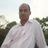Secondary Computer & Science Teacher um Teaching Computer Science in PSBS, Kirtipur
28. Feb 2014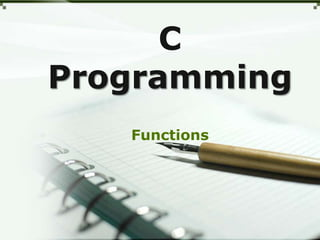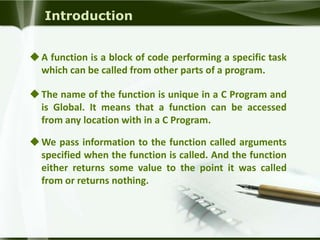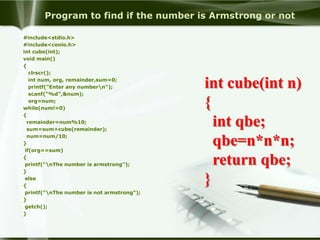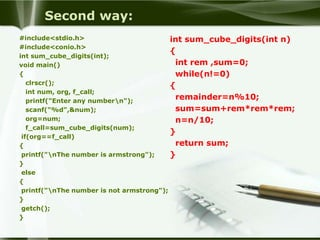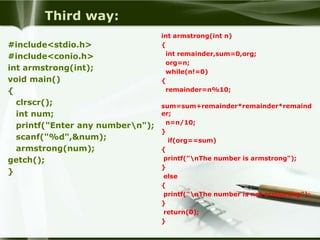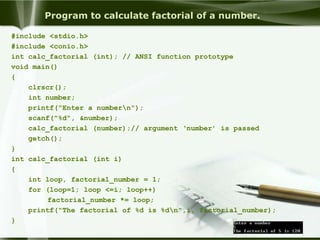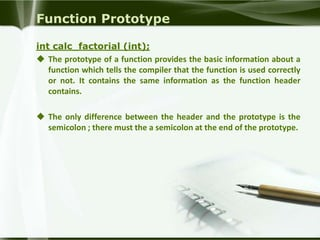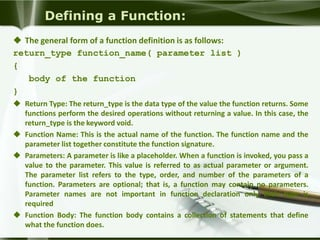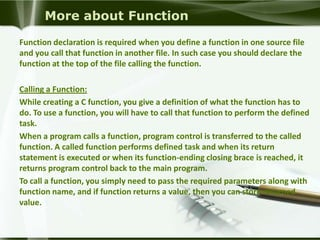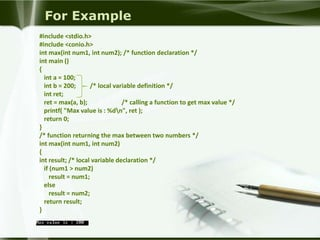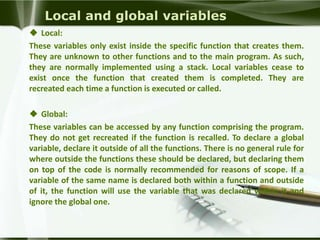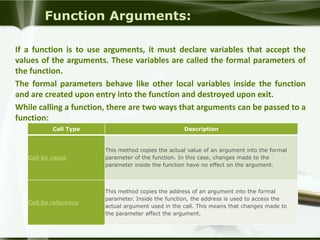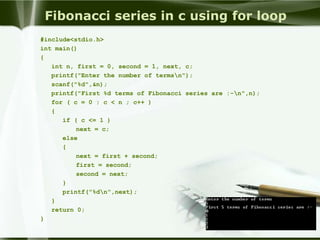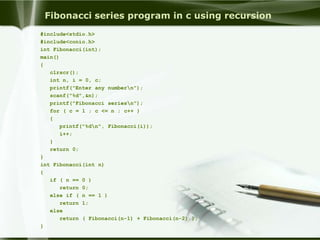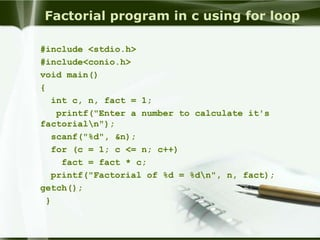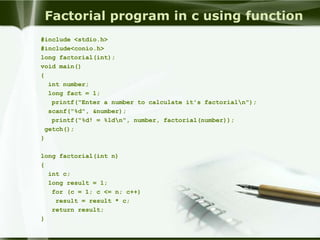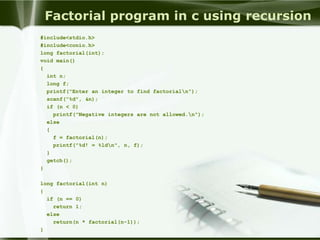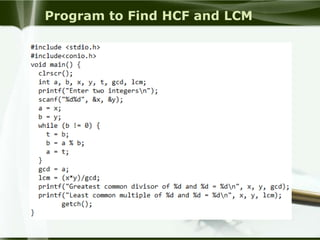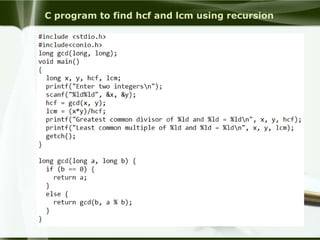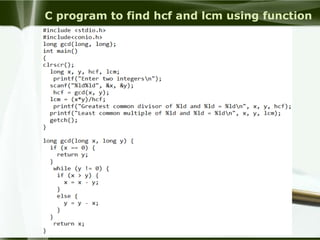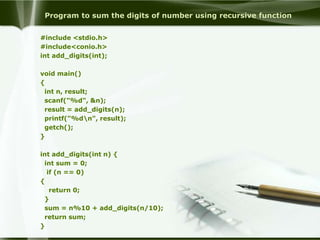1 von 22

### Function in c

• 2. Introduction  A function is a block of code performing a specific task which can be called from other parts of a program.  The name of the function is unique in a C Program and is Global. It means that a function can be accessed from any location with in a C Program.  We pass information to the function called arguments specified when the function is called. And the function either returns some value to the point it was called from or returns nothing.
• 3. Program to find if the number is Armstrong or not #include<stdio.h> #include<conio.h> int cube(int); void main() { clrscr(); int num, org, remainder,sum=0; printf("Enter any numbern"); scanf("%d",&num); org=num; while(num!=0) { remainder=num%10; sum=sum+cube(remainder); num=num/10; } if(org==sum) { printf("nThe number is armstrong"); } else { printf("nThe number is not armstrong"); } getch(); } int cube(int n) { int qbe; qbe=n*n*n; return qbe; }
• 4. Second way: #include<stdio.h> int sum_cube_digits(int n) #include<conio.h> { int sum_cube_digits(int); int rem ,sum=0; void main() { while(n!=0) clrscr(); { int num, org, f_call; remainder=n%10; printf("Enter any numbern"); sum=sum+rem*rem*rem; scanf("%d",&num); org=num; n=n/10; f_call=sum_cube_digits(num); } if(org==f_call) return sum; { printf("nThe number is armstrong"); } } else { printf("nThe number is not armstrong"); } getch(); }
• 5. Third way: #include<stdio.h> #include<conio.h> int armstrong(int); void main() { clrscr(); int num; printf("Enter any numbern"); scanf("%d",&num); armstrong(num); getch(); } int armstrong(int n) { int remainder,sum=0,org; org=n; while(n!=0) { remainder=n%10; sum=sum+remainder*remainder*remaind er; n=n/10; } if(org==sum) { printf("nThe number is armstrong"); } else { printf("nThe number is not armstrong"); } return(0); }
• 6. Program to calculate factorial of a number. #include <stdio.h> #include <conio.h> int calc_factorial (int); // ANSI function prototype void main() { clrscr(); int number; printf("Enter a numbern"); scanf("%d", &number); calc_factorial (number);// argument ‘number’ is passed getch(); } int calc_factorial (int i) { int loop, factorial_number = 1; for (loop=1; loop <=i; loop++) factorial_number *= loop; printf("The factorial of %d is %dn",i, factorial_number); }
• 7. Function Prototype int calc_factorial (int);  The prototype of a function provides the basic information about a function which tells the compiler that the function is used correctly or not. It contains the same information as the function header contains.  The only difference between the header and the prototype is the semicolon ; there must the a semicolon at the end of the prototype.
• 8. Defining a Function:  The general form of a function definition is as follows: return_type function_name( parameter list ) { body of the function }  Return Type: The return_type is the data type of the value the function returns. Some functions perform the desired operations without returning a value. In this case, the return_type is the keyword void.  Function Name: This is the actual name of the function. The function name and the parameter list together constitute the function signature.  Parameters: A parameter is like a placeholder. When a function is invoked, you pass a value to the parameter. This value is referred to as actual parameter or argument. The parameter list refers to the type, order, and number of the parameters of a function. Parameters are optional; that is, a function may contain no parameters. Parameter names are not important in function declaration only their type is required  Function Body: The function body contains a collection of statements that define what the function does.
• 9. More about Function Function declaration is required when you define a function in one source file and you call that function in another file. In such case you should declare the function at the top of the file calling the function. Calling a Function: While creating a C function, you give a definition of what the function has to do. To use a function, you will have to call that function to perform the defined task. When a program calls a function, program control is transferred to the called function. A called function performs defined task and when its return statement is executed or when its function-ending closing brace is reached, it returns program control back to the main program. To call a function, you simply need to pass the required parameters along with function name, and if function returns a value, then you can store returned value.
• 10. For Example #include <stdio.h> #include <conio.h> int max(int num1, int num2); /* function declaration */ int main () { int a = 100; int b = 200; /* local variable definition */ int ret; ret = max(a, b); /* calling a function to get max value */ printf( "Max value is : %dn", ret ); return 0; } /* function returning the max between two numbers */ int max(int num1, int num2) { int result; /* local variable declaration */ if (num1 > num2) result = num1; else result = num2; return result; }
• 11. Local and global variables  Local: These variables only exist inside the specific function that creates them. They are unknown to other functions and to the main program. As such, they are normally implemented using a stack. Local variables cease to exist once the function that created them is completed. They are recreated each time a function is executed or called.  Global: These variables can be accessed by any function comprising the program. They do not get recreated if the function is recalled. To declare a global variable, declare it outside of all the functions. There is no general rule for where outside the functions these should be declared, but declaring them on top of the code is normally recommended for reasons of scope. If a variable of the same name is declared both within a function and outside of it, the function will use the variable that was declared within it and ignore the global one.
• 12. Function Arguments: If a function is to use arguments, it must declare variables that accept the values of the arguments. These variables are called the formal parameters of the function. The formal parameters behave like other local variables inside the function and are created upon entry into the function and destroyed upon exit. While calling a function, there are two ways that arguments can be passed to a function: Call Type Description Call by value This method copies the actual value of an argument into the formal parameter of the function. In this case, changes made to the parameter inside the function have no effect on the argument. Call by reference This method copies the address of an argument into the formal parameter. Inside the function, the address is used to access the actual argument used in the call. This means that changes made to the parameter affect the argument.
• 13. Fibonacci series in c using for loop #include<stdio.h> int main() { int n, first = 0, second = 1, next, c; printf("Enter the number of termsn"); scanf("%d",&n); printf("First %d terms of Fibonacci series are :-n",n); for ( c = 0 ; c < n ; c++ ) { if ( c <= 1 ) next = c; else { next = first + second; first = second; second = next; } printf("%dn",next); } return 0; }
• 14. Fibonacci series program in c using recursion #include<stdio.h> #include<conio.h> int Fibonacci(int); main() { clrscr(); int n, i = 0, c; printf("Enter any numbern"); scanf("%d",&n); printf("Fibonacci seriesn"); for ( c = 1 ; c <= n ; c++ ) { printf("%dn", Fibonacci(i)); i++; } return 0; } int Fibonacci(int n) { if ( n == 0 ) return 0; else if ( n == 1 ) return 1; else return ( Fibonacci(n-1) + Fibonacci(n-2) ); }
• 15. Factorial program in c using for loop #include <stdio.h> #include<conio.h> void main() { int c, n, fact = 1; printf("Enter a number to calculate it's factorialn"); scanf("%d", &n); for (c = 1; c <= n; c++) fact = fact * c; printf("Factorial of %d = %dn", n, fact); getch(); }
• 16. Factorial program in c using function #include <stdio.h> #include<conio.h> long factorial(int); void main() { int number; long fact = 1; printf("Enter a number to calculate it's factorialn"); scanf("%d", &number); printf("%d! = %ldn", number, factorial(number)); getch(); } long factorial(int n) { int c; long result = 1; for (c = 1; c <= n; c++) result = result * c; return result; }
• 17. Factorial program in c using recursion #include<stdio.h> #include<conio.h> long factorial(int); void main() { int n; long f; printf("Enter an integer to find factorialn"); scanf("%d", &n); if (n < 0) printf("Negative integers are not allowed.n"); else { f = factorial(n); printf("%d! = %ldn", n, f); } getch(); } long factorial(int n) { if (n == 0) return 1; else return(n * factorial(n-1)); }
• 18. Program to Find HCF and LCM
• 19. C program to find hcf and lcm using recursion
• 20. C program to find hcf and lcm using function
• 21. Program to sum the digits of number using recursive function #include <stdio.h> #include<conio.h> int add_digits(int); void main() { int n, result; scanf("%d", &n); result = add_digits(n); printf("%dn", result); getch(); } int add_digits(int n) { int sum = 0; if (n == 0) { return 0; } sum = n%10 + add_digits(n/10); return sum; }
• 22. Thank You Raj Kumar Tandukar rktandukar@hotmail.com